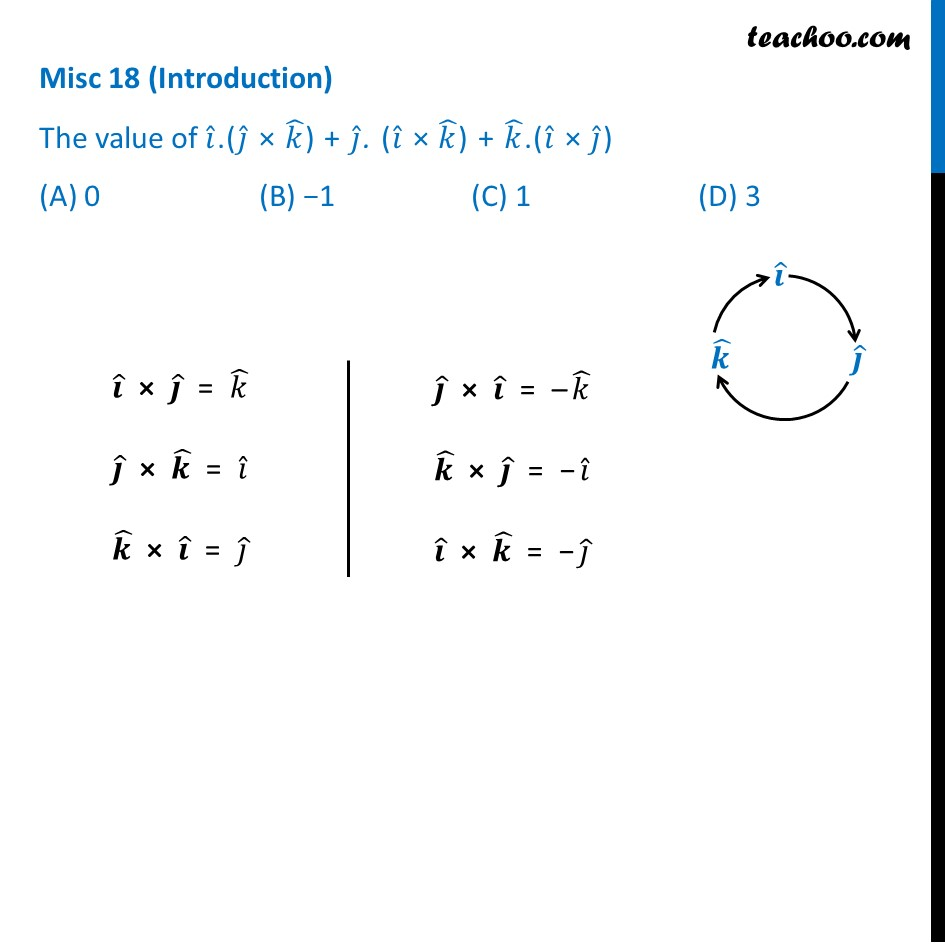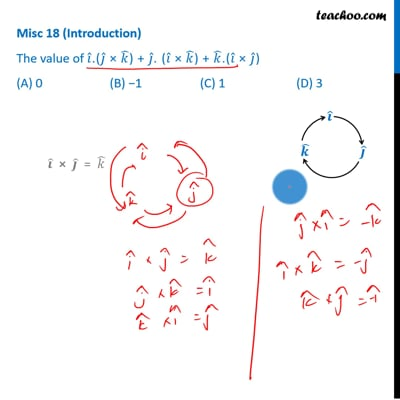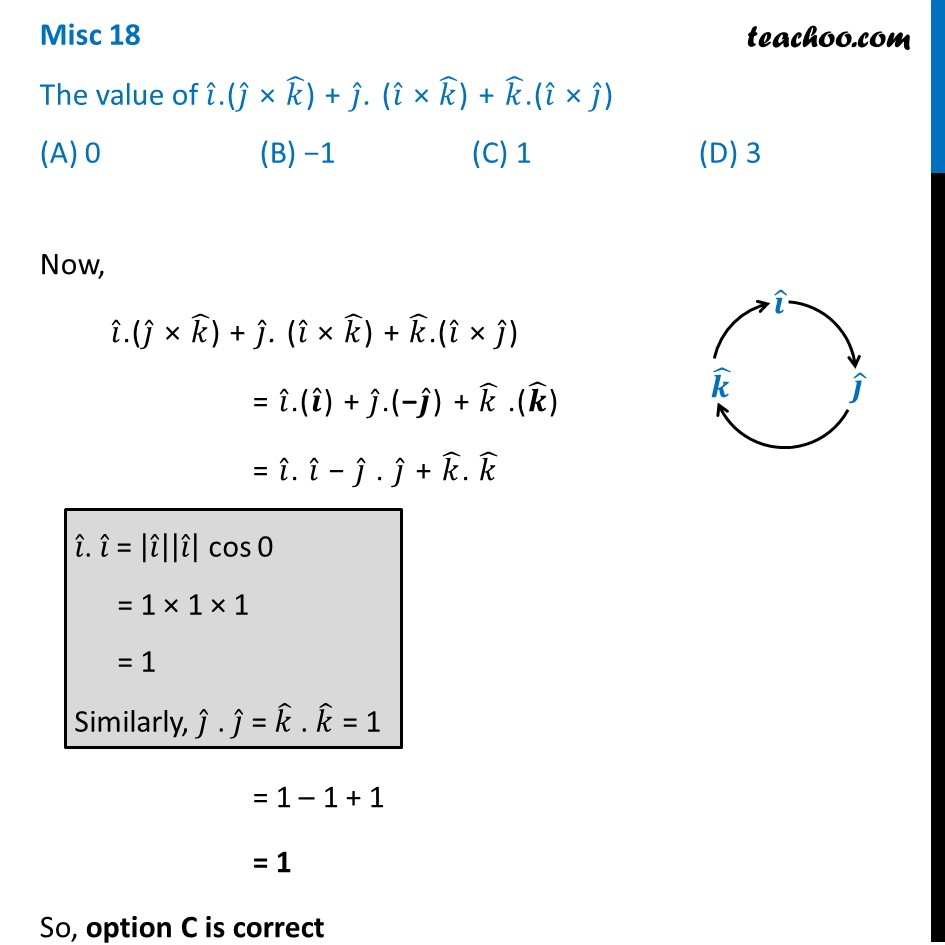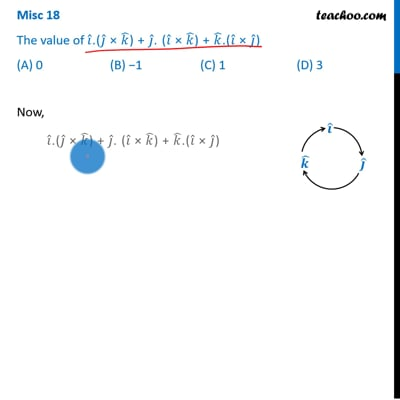Miscellaneous

Chapter 10 Class 12 Vector Algebra
Serial order wiseThis video is only available for Teachoo black usersThis video is only available for Teachoo black users

Solve all your doubts with Teachoo Black (new monthly pack available now!)

### Transcript

Misc 18 (Introduction) The value of 𝑖 ̂.(𝑗 ̂ × 𝑘 ̂) + 𝑗 ̂. (𝑖 ̂ × 𝑘 ̂) + 𝑘 ̂.(𝑖 ̂ × 𝑗 ̂) (A) 0 (B) −1 (C) 1 (D) 3 𝒊 ̂ × 𝒋 ̂ = 𝑘 ̂ 𝒋 ̂ × 𝒌 ̂ = 𝑖 ̂ 𝒌 ̂ × 𝒊 ̂ = 𝑗 ̂ 𝒋 ̂ × 𝒊 ̂ = –𝑘 ̂ 𝒌 ̂ × 𝒋 ̂ = −𝑖 ̂ 𝒊 ̂ × 𝒌 ̂ = −𝑗 ̂ Misc 18 The value of 𝑖 ̂.(𝑗 ̂ × 𝑘 ̂) + 𝑗 ̂. (𝑖 ̂ × 𝑘 ̂) + 𝑘 ̂.(𝑖 ̂ × 𝑗 ̂) (A) 0 (B) −1 (C) 1 (D) 3 Now, 𝑖 ̂.(𝑗 ̂ × 𝑘 ̂) + 𝑗 ̂. (𝑖 ̂ × 𝑘 ̂) + 𝑘 ̂.(𝑖 ̂ × 𝑗 ̂) = 𝑖 ̂.(𝒊 ̂) + 𝑗 ̂.(−𝒋 ̂) + 𝑘 ̂ .(𝒌 ̂) = 𝑖 ̂. 𝑖 ̂ − 𝑗 ̂ . 𝑗 ̂ + 𝑘 ̂. 𝑘 ̂ = 1 – 1 + 1 = 1 So, option C is correct 𝑖 ̂. 𝑖 ̂ = |𝑖 ̂ ||𝑖 ̂ | cos 0 = 1 × 1 × 1 = 1 Similarly, 𝑗 ̂ . 𝑗 ̂ = 𝑘 ̂ . 𝑘 ̂ = 1